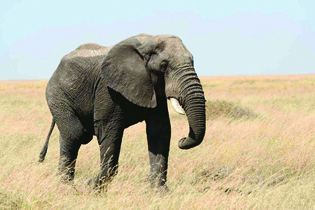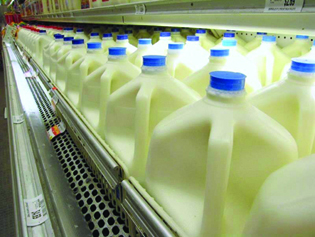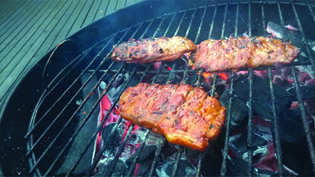## Making Unit Conversions in the U.S. System of Measurement

### Learning Outcomes

• Make unit conversions in the U.S. system
• Use mixed units of measurement in the U.S. system

There are two systems of measurement commonly used around the world. Most countries use the metric system. The United States uses a different system of measurement, usually called the U.S. system. We will look at the U.S. system first.

The U.S. system of measurement uses units of inch, foot, yard, and mile to measure length and pound and ton to measure weight. For capacity, the units used are cup, pint, quart and gallons. Both the U.S. system and the metric system measure time in seconds, minutes, or hours.

The equivalencies among the basic units of the U.S. system of measurement are listed in the table below. The table also shows, in parentheses, the common abbreviations for each measurement.

U.S. System Units
Length Volume
$1$ foot (ft) = $12$ inches (in)

$1$ yard (yd) = $3$ feet (ft)

$1$ mile (mi) = $5280$ feet (ft)

$3$ teaspoons (t) = $1$ tablespoon (T)

$16$ Tablespoons (T) = $1$ cup (C)

$1$ cup (C) = $8$ fluid ounces (fl oz)

$1$ pint (pt) = $2$ cups (C)

$1$ quart (qt) = $2$ pints (pt)

$1$ gallon (gal) = $4$ quarts (qt)

Weight Time
$1$ pound (lb) = $16$ ounces (oz)

$1$ ton = $2000$ pounds (lb)

$1$ minute (min) = $60$ seconds (s)

$1$ hour (h) = $60$ minutes (min)

$1$ day = $24$ hours (h)

$1$ week (wk) = $7$ days

$1$ year (yr) = $365$ days

In many real-life applications, we need to convert between units of measurement. We will use the identity property of multiplication to do these conversions. We’ll restate the Identity Property of Multiplication here for easy reference.

$\text{For any real number }a,a\cdot 1=a$    $1\cdot a=a$

To use the identity property of multiplication, we write $1$ in a form that will help us convert the units. For example, suppose we want to convert inches to feet. We know that $1$ foot is equal to $12$ inches, so we can write $1$ as the fraction $\Large\frac{\text{1 ft}}{\text{12 in}}$. When we multiply by this fraction, we do not change the value but just change the units.

But $\Large\frac{\text{12 in}}{\text{1 ft}}$ also equals $1$. How do we decide whether to multiply by $\Large\frac{\text{1 ft}}{\text{12 in}}$ or $\Large\frac{\text{12 in}}{\text{1 ft}}?$ We choose the fraction that will make the units we want to convert from divide out. For example, suppose we wanted to convert $60$ inches to feet. If we choose the fraction that has inches in the denominator, we can eliminate the inches.

$60\overline{)\text{in}}\cdot\Large\frac{\text{1 ft}}{12\overline{)\text{in}}}\normalsize=\text{5 ft}$

On the other hand, if we wanted to convert $5$ feet to inches, we would choose the fraction that has feet in the denominator.

$\text{5 ft}\cdot\Large\frac{\text{12 in}}{1\overline{)\text{ft}}}\normalsize=\text{60 in}$

We treat the unit words like factors and ‘divide out’ common units like we do common factors.

### Make unit conversions.

1. Multiply the measurement to be converted by $1$; write $1$ as a fraction relating the units given and the units needed.
2. Multiply.
3. Simplify the fraction, performing the indicated operations and removing the common units.

### example

Mary Anne is $66$ inches tall. What is her height in feet?

Solution

 Convert $66$ inches into feet. Multiply the measurement to be converted by $1$. $66$ inches $\cdot 1$ Write $1$ as a fraction relating the units given and the units needed. $\text{66 inches}\cdot \Large\frac{\text{1 foot}}{\text{12 inches}}$ Multiply. $\Large\frac{\text{66 inches}\cdot \text{1 foot}}{\text{12 inches}}$ Simplify the fraction. $\Large\frac{66\overline{)\text{inches}}\cdot \text{1 foot}}{12\overline{)\text{inches}}}$ $\Large\frac{\text{66 feet}}{12}$ $\text{5.5 feet}$

Notice that when we simplified the fraction, we first divided out the inches.
Mary Anne is $5.5$ feet tall.

### try it

In the following video we show more examples of converting between units of length in the U.S. system of measurement.

When we use the Identity Property of Multiplication to convert units, we need to make sure the units we want to change from will divide out. Usually this means we want the conversion fraction to have those units in the denominator.

### example

Ndula, an elephant at the San Diego Safari Park, weighs almost $3.2$ tons. Convert her weight to pounds.

(credit: Guldo Da Rozze, Flickr)### try it

In  the next video we show more examples of how to convert between units of weight using the U. S. system of measurement.

Sometimes to convert from one unit to another, we may need to use several other units in between, so we will need to multiply several fractions.

### example

Juliet is going with her family to their summer home. She will be away for $9$ weeks. Convert the time to minutes.

### try it

The distance between Earth and the moon is about $250,000$ miles. Convert this length to yards.

A team of astronauts spends $15$ weeks in space. Convert the time to minutes.

### example

How many fluid ounces are in $1$ gallon of milk?

(credit: www.bluewaikiki.com, Flickr)### try it

How many teaspoons are in $1$ cup?

### Use Mixed Units of Measurement in the U.S. System

Performing arithmetic operations on measurements with mixed units of measures requires care. Be sure to add or subtract like units.

### example

Charlie bought three steaks for a barbecue. Their weights were $14$ ounces, $1$ pound $2$ ounces, and $1$ pound $6$ ounces. How many total pounds of steak did he buy?

(credit: Helen Penjam, Flickr)### example

Anthony bought four planks of wood that were each $6$ feet $4$ inches long. If the four planks are placed end-to-end, what is the total length of the wood?## Contribute!

Did you have an idea for improving this content? We’d love your input.